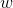# Question A block of mass m = 0.5 kg is attached to a horizontal spring whose spring constant is k = 50 N/m. At t = 0.1s, the position is x = -0.2 m and the velocity is vx = +0.5 m/s. It is assumed that x(t) = A sin(t+ φ). (a) Determine the amplitude and phase constant. (b) At what time does the condition x = 0.2 m and vx= -0.5 m/s first occur?A block of mass m = 0.5 kg is attached to a horizontal spring whose spring constant is k = 50 N/m. At t = 0.1s, the position is x = -0.2 m and the velocity is vx = +0.5 m/s. It is assumed that x(t) = A sin(t+ φ). (a) Determine the amplitude and phase constant. (b) At what time does the condition x = 0.2 m and vx= -0.5 m/s first occur?